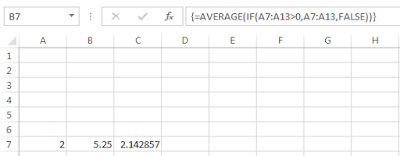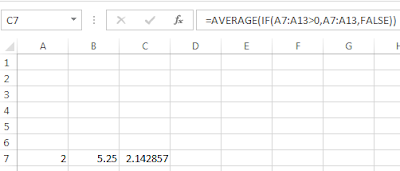## Monday, 29 August 2016

### Arrays vs. Arrayformulae

I find myself having to defend my intense dislike of Excel's array formulae methodology. Since I’m no expert in this aspect of Excel, this consideration relies upon Chip Pearson’s advice.

In reality, most familiar Excel formulae use arrays like =SUM(A2:A4). In this case the formula instructs Excel to sum all the values in the array of cells {A2, A3, A4}. In fact the formula = SUM(A2, A3, A4)  performs exactly the same task as =SUM(A2:A4).  Excel is also quite happy mixing different forms of presentation such as =SUM(A2:A4, C5:C20, D1:D2). In short Excel is pretty clever at interpreting user requirements.

Array formulae are different and the user must give Excel a specific instruction for the software to handle the instruction correctly. This takes the form of curly braces around the formulae {} generated by posting the formula using Control, Shift and Enter.

Chip offers the example of {=AVERAGE(IF(A7:A13>0,A7:A13,FALSE))}. Interpret this in plain English as for each element in the array A7:A13, if it is more than zero include it is the average otherwise leave it out (FALSE). The curly braces are absolutely crucial to this interpretation. Here is the evaluation of this formula with the curly braces in place.See cell B7 for the result 5.25
On the other hand the identical formula, if posted using Enter it delivers the following result. This is a standard average.See cell C7 for the result 2.142857

Even more confusing, if you list the formulae in your worksheet by evaluating each cell for its formula you get the following. Here it looks as if the identical formula cell delivers different results, because formula is never listed with its curly braces.

To me this was sufficient to put me off ever using an array formula, but in reality this is the more simple methodology.

You can use array formulae to deliver an array of answers. The spreadsheet below has identical formulae in each of cells in columns E {=ROW(A7:A15)} and similarly in column  F {=A7:A13}. However, because they are entered using the control, shift method each cell delivers a different answer appropriate to its position in the array.Arrayformuylae answers delivered according to their position in the array
If, you list the formulae however it is not possible to tell that these have been array entered – as shown below. The user or a reviewer has to deduce the array entry methodology based on the number of identical formulae which deliver different answers.In each of these cases the identical formula appears to delivers a series of different answers
In all other languages that I have used, it has always been immediately obvious when working with arrays. It simplifies matters both for the user and the auditor.

No matter how technically clever Excel’s array formula methodology, I will always avoid it if I can.